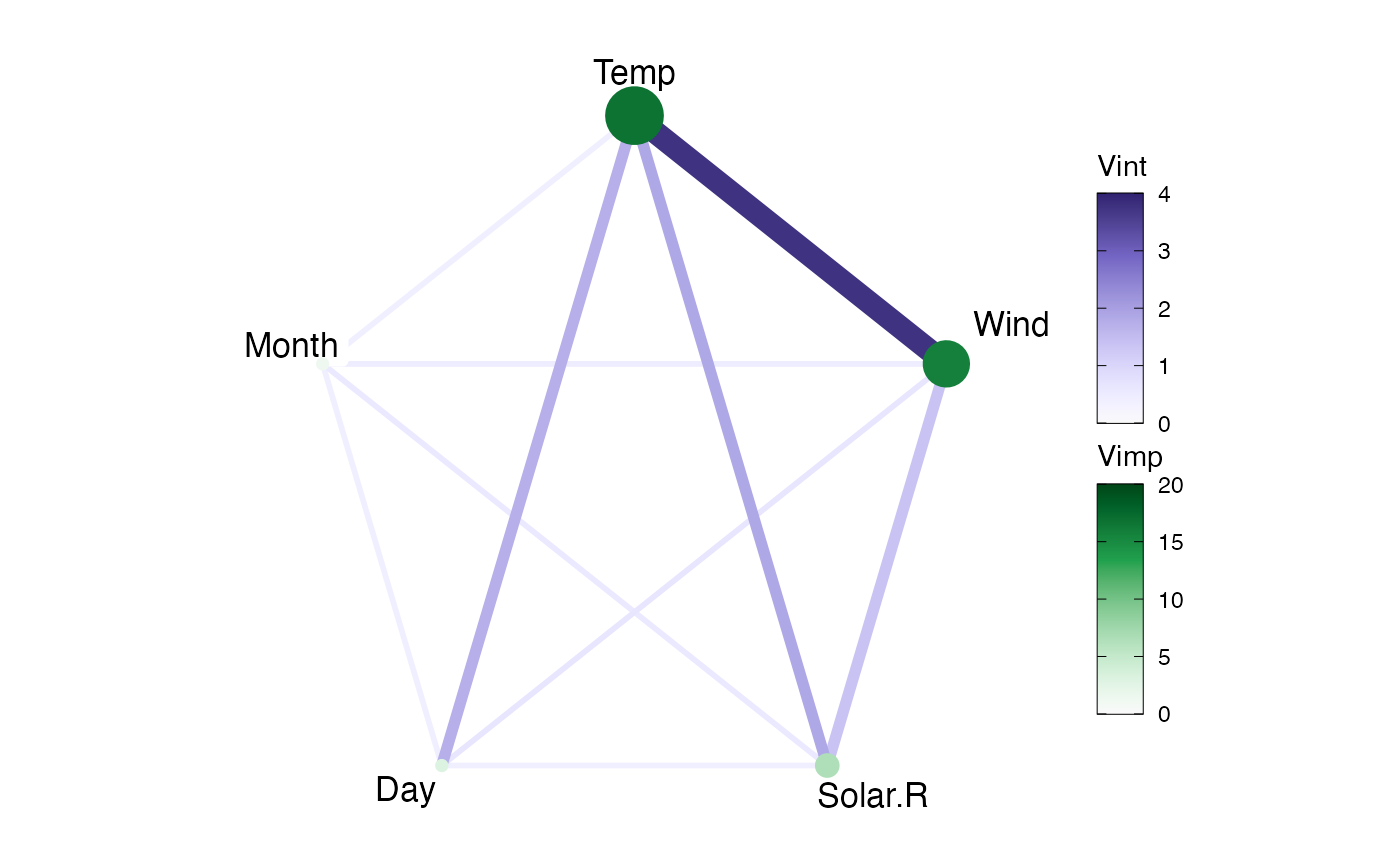Create a Network plot displaying variable importance and variable interaction.

viviNetwork(
mat,
intThreshold = NULL,
intLims = NULL,
impLims = NULL,
intPal = rev(colorspace::sequential_hcl(palette = "Purples 3", n = 100)),
impPal = rev(colorspace::sequential_hcl(palette = "Greens 3", n = 100)),
removeNode = FALSE,
layout = igraph::layout_in_circle,
cluster = NULL,
nudge_x = 0.05,
nudge_y = 0.03,
edgeWidths = 1:4
)

## Arguments

mat A matrix, such as that returned by vivi, of values to be plotted. Remove edges with weight below this value if provided. Specifies the fit range for the color map for interaction strength. Specifies the fit range for the color map for importance. A vector of colours to show interactions, for use with scale_fill_gradientn. A vector of colours to show importance, for use with scale_fill_gradientn. If TRUE, then removes nodes with no connecting edges when thresholding interaction values. igraph layout function or a numeric matrix with two columns, one row per node. Defaults to igraph::layout_as_circle Either a vector of cluster memberships for nodes or an igraph clustering function. Nudge (centered) labels by this amount, outward horizontally. Nudge (centered) labels by this amount, outward vertically. A vector specifying the scaling of the edges for the displayed graph. Values must be positive.

## Value

A plot displaying interaction strength between variables on the edges and variable importance on the nodes.

## Examples

# \donttest{
library(ranger)
aq <- na.omit(airquality)
rF <- ranger(Ozone ~ ., data = aq, importance = "permutation")
myMat <- vivi(fit = rF, data = aq, response = "Ozone")
#> Agnostic variable importance method used.
#> Calculating interactions...
viviNetwork(myMat)# }